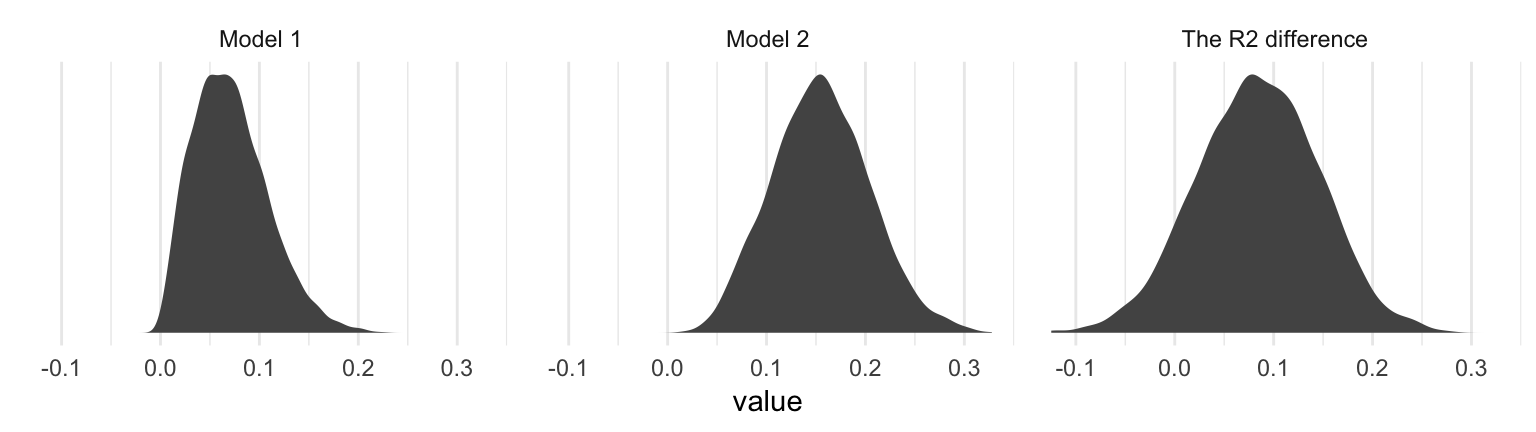## 10.2 An example from the sex disrimination in the workplace study

Here we load a couple necessary packages, load the data, and take a glimpse().

library(tidyverse)

protest <- read_csv("data/protest/protest.csv")

glimpse(protest)
## Observations: 129
## Variables: 6
## $subnum <int> 209, 44, 124, 232, 30, 140, 27, 64, 67, 182, 85, 109, 122, 69, 45, 28, 170, 66... ##$ protest  <int> 2, 0, 2, 2, 2, 1, 2, 0, 0, 0, 2, 2, 0, 1, 1, 0, 1, 2, 2, 1, 2, 1, 1, 2, 2, 0, ...
## $sexism <dbl> 4.87, 4.25, 5.00, 5.50, 5.62, 5.75, 5.12, 6.62, 5.75, 4.62, 4.75, 6.12, 4.87, ... ##$ angry    <int> 2, 1, 3, 1, 1, 1, 2, 1, 6, 1, 2, 5, 2, 1, 1, 1, 2, 1, 3, 4, 1, 1, 1, 5, 1, 5, ...
## $liking <dbl> 4.83, 4.50, 5.50, 5.66, 6.16, 6.00, 4.66, 6.50, 1.00, 6.83, 5.00, 5.66, 5.83, ... ##$ respappr <dbl> 4.25, 5.75, 4.75, 7.00, 6.75, 5.50, 5.00, 6.25, 3.00, 5.75, 5.25, 7.00, 4.50, ...

With a little ifelse(), computing the dummies D1 and D2 is easy enough.

protest <-
protest %>%
mutate(D1 = ifelse(protest == 1, 1, 0),
D2 = ifelse(protest == 2, 1, 0))

Load brms.

library(brms)

With model1 and model2 we fit the multicategorical multivariable model and the multicategorical moderation models, respectively.

model1 <-
brm(data = protest, family = gaussian,
liking ~ 1 + D1 + D2 + sexism,
chains = 4, cores = 4)

model2 <-
update(model1,
newdata = protest,
liking ~ 1 + D1 + D2 + sexism + D1:sexism + D2:sexism,
chains = 4, cores = 4)

Behold the $$R^2$$s.

r2s <-
bayes_R2(model1, summary = F) %>%
as_tibble() %>%
rename(Model 1 = R2) %>%
bind_cols(
bayes_R2(model2, summary = F) %>%
as_tibble() %>%
rename(Model 2 = R2)
) %>%
mutate(The R2 difference = Model 2 - Model 1)

r2s %>%
gather()  %>%
# This line isn't necessary, but it sets the order the summaries appear in
mutate(key = factor(key, levels = c("Model 1", "Model 2", "The R2 difference"))) %>%
group_by(key) %>%
summarize(mean = mean(value),
median = median(value),
ll = quantile(value, probs = .025),
ul = quantile(value,  probs = .975)) %>%
mutate_if(is.double, round, digits = 3)
## # A tibble: 3 x 5
##   key                mean median     ll    ul
##   <fct>             <dbl>  <dbl>  <dbl> <dbl>
## 1 Model 1           0.071  0.067  0.012 0.156
## 2 Model 2           0.155  0.154  0.064 0.256
## 3 The R2 difference 0.084  0.084 -0.039 0.202

Interestingly, even though our posterior means and medians for the model-specific $$R^2$$ values differed some from the OLS estimates in the text, their difference corresponded quite nicely to the one in the text. Let’s take a look at their distributions.

r2s %>%
gather() %>%
ggplot(aes(x = value)) +
geom_density(size = 0, fill = "grey33") +
scale_y_continuous(NULL, breaks = NULL) +
facet_wrap(~key, scales = "free_y") +
theme_minimal()The model coefficient summaries cohere well with those in Table 10.1.

print(model1, digits = 3)
##  Family: gaussian
##   Links: mu = identity; sigma = identity
## Formula: liking ~ 1 + D1 + D2 + sexism
##    Data: protest (Number of observations: 129)
## Samples: 4 chains, each with iter = 2000; warmup = 1000; thin = 1;
##          total post-warmup samples = 4000
##
## Population-Level Effects:
##           Estimate Est.Error l-95% CI u-95% CI Eff.Sample  Rhat
## Intercept    4.749     0.619    3.557    5.962       4000 1.000
## D1           0.497     0.233    0.036    0.958       4000 1.000
## D2           0.448     0.227    0.003    0.891       4000 1.000
## sexism       0.110     0.118   -0.118    0.340       4000 1.000
##
## Family Specific Parameters:
##       Estimate Est.Error l-95% CI u-95% CI Eff.Sample  Rhat
## sigma    1.044     0.067    0.918    1.184       4000 0.999
##
## Samples were drawn using sampling(NUTS). For each parameter, Eff.Sample
## is a crude measure of effective sample size, and Rhat is the potential
## scale reduction factor on split chains (at convergence, Rhat = 1).
print(model2, digits = 3)
##  Family: gaussian
##   Links: mu = identity; sigma = identity
## Formula: liking ~ D1 + D2 + sexism + D1:sexism + D2:sexism
##    Data: protest (Number of observations: 129)
## Samples: 4 chains, each with iter = 2000; warmup = 1000; thin = 1;
##          total post-warmup samples = 4000
##
## Population-Level Effects:
##           Estimate Est.Error l-95% CI u-95% CI Eff.Sample  Rhat
## Intercept    7.709     1.055    5.721    9.828       1502 1.001
## D1          -4.110     1.522   -7.057   -1.093       1551 1.000
## D2          -3.498     1.429   -6.246   -0.722       1415 1.001
## sexism      -0.473     0.206   -0.889   -0.077       1485 1.001
## D1:sexism    0.897     0.291    0.319    1.462       1524 1.001
## D2:sexism    0.778     0.279    0.239    1.318       1406 1.001
##
## Family Specific Parameters:
##       Estimate Est.Error l-95% CI u-95% CI Eff.Sample  Rhat
## sigma    1.006     0.064    0.890    1.143       2865 1.000
##
## Samples were drawn using sampling(NUTS). For each parameter, Eff.Sample
## is a crude measure of effective sample size, and Rhat is the potential
## scale reduction factor on split chains (at convergence, Rhat = 1).